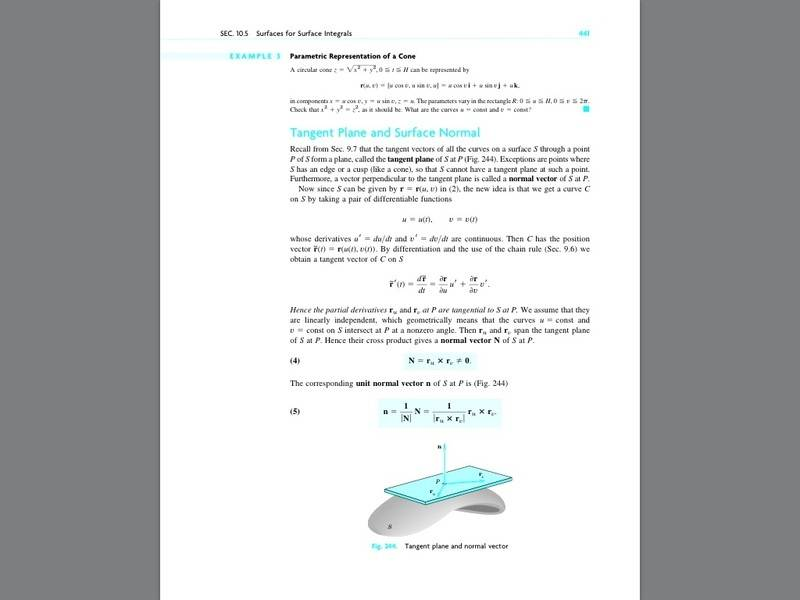# "Hence the partial derivatives ru and rv at P are tangential

• I
I've been looking at the equation for r tilde prime in the image I attached below, but I cannot understand why it is that they say "Hence, the partial derivatives ru and rv at P are tangential to S at P".How does that equation imply that ru and rv are tangential to P?

•